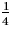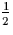Next: A recursive definition Up: Limits within [-1,1] Previous: Determining output digits

### Input digits required

We now show that we need to examine at most three input digits from x and z in order to either determine at least one additional output digit, or to determine that the lower and upper bounds are definitely not equal. In doing so we satisfy the conditions given in section 5.1.

After examining three digits of the input, we know that the range of both the lower and upper bounds is of width. If the lower and upper bounds are not disjoint, the width of the range of possible numbers which fall between the least possible lower bound and the greatest possible upper bound is not greater than.

Given any interval whose width is less than or equal to, we can find a single digit whose range encloses the interval. We can also say that if there exists no signed binary digit containing both intervals of width, then the intervals are definitely disjoint.

Martin Escardo
5/11/2000